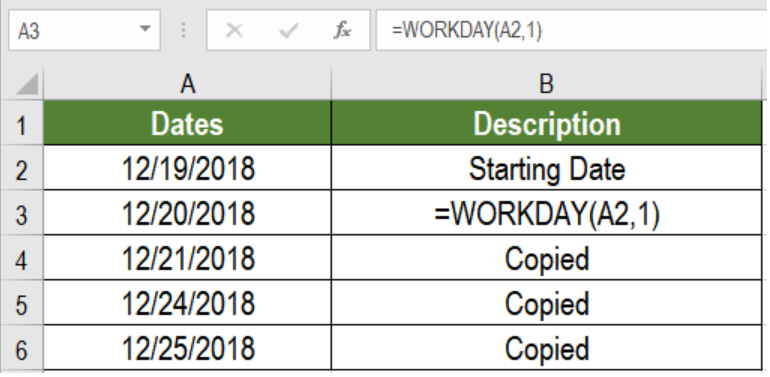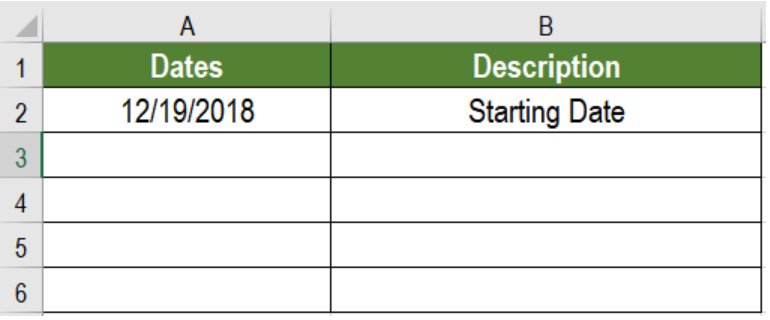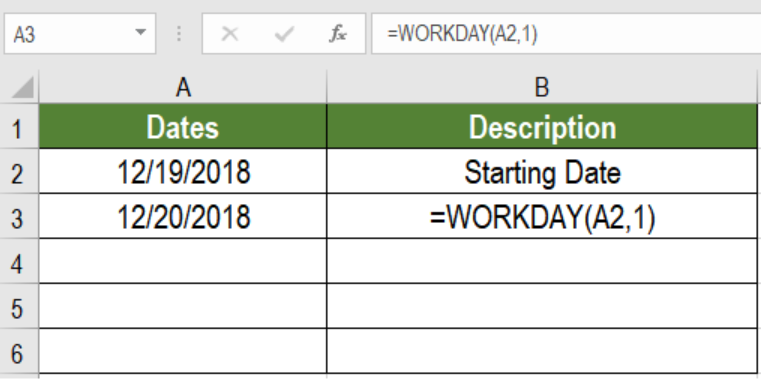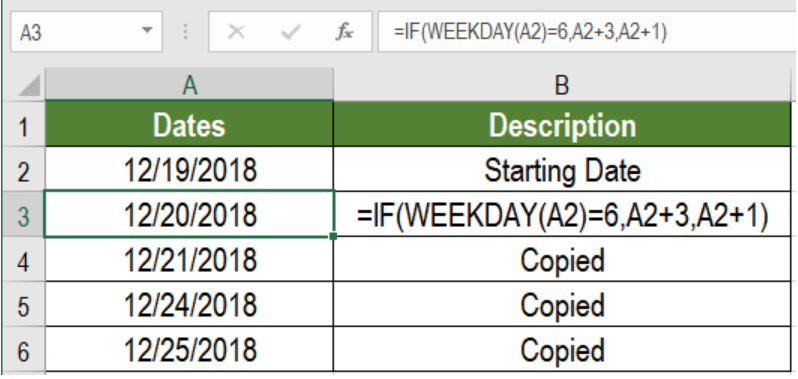Get instant live expert help with Excel or Google Sheets“My Excelchat expert helped me in less than 20 minutes, saving me what would have been 5 hours of work!”

#### Post your problem and you’ll get Expert help in seconds.

Your message must be at least 40 characters
Our professional Expert are available now. Your privacy is guaranteed.

# How to Generate a Series of Dates by Workdays in Excel

Working with dates is one of the most challenging aspects for many. However, Excel has made working with dates simpler over time. One of these features offered by Excel is to generate weekdays excluding weekends. We can do it using the WORKDAY function. In this tutorial, we will be learn how to get a series of dates by workdays in Excel.Figure 1. Example of How to Get a Series of Dates by Workdays

## Generic Formula

`=WORKDAY(start_date,days,[holidays])`

## How the Function Works

Here, we are using the WORKDAY function. It extracts the next working date from the date provided. The arguments used are:

• start_date – This argument is required. It is the starting day from which WORKDAY starts generating dates.
• days – This argument is also required. We will use 1 here. It represents the code of weekend dates. 1 stands for Saturday and Sunday.
• [holidays] – This one argument is optional. It represents the non-working dates. Such as, federal or floating holidays.

The WORKDAY function extracts the next working day from the starting date. We can provide custom holidays to skip in [holidays] argument. This will skip the dates for those days.

## Setting up Data

We will be using a data set with a starting date in column A.Figure 2. The Sample Data Set

To generate a series of dates by workdays, we need to:

• Go to cell A3.
• Assign the formula `=WORKDAY(A2,1) `to A3.
• Press Enter.
• Drag the formula from cells A3 to A6.Figure 3. Applying the Formula to the Data

After completing all this we will get a series of dates by workdays.

## Note

• If you have different weekends than Saturday and Sunday, you need to customize the day. The default provides 1.
• You can solve the previous example by the WEEKDAY function. We need to assign the formula =IF(WEEKDAY(A2)=6,A2+3,A2+1) to A3.Figure 4. Example of Using the WEEKDAY Function

Most of the time, the problem you will need to solve will be more complex than a simple application of a formula or function. If you want to save hours of research and frustration, try our live Excelchat service! Our Excel Experts are available 24/7 to answer any Excel question you may have. We guarantee a connection within 30 seconds and a customized solution within 20 minutes.

### Did this post not answer your question? Get a solution from connecting with the expert.Another blog reader asked this question today on Excelchat:
Related blogs
Solution examplesI need to create a formula where column D becomes the date in column C + 5 business days when I enter a date into column C
Solved by X. Q. in 14 minsneed help with the different sheets and formula IF(A4=B4,0,NETWORKDAYS(A4+1,B4,\$E\$9:\$E\$42) +0.5*(INT((+B4-A4+1)/7)+IF(WEEKDAY(A4+1)+MOD (B4-A4-1,7)>=7,1,0)))
Solved by O. D. in 11 minsi have this formula currently which works but not if I want it for every day - any ideas ?? (NETWORKDAYS.INTL(L1362,M1362,11,BH!A:A)-1)*("17:30"-"8:30")+IF(NETWORKDAYS.INTL(M1362,M1362,11,BH!A:A),MEDIAN(MOD(M1362,1),"8:30","17:30"),"17:30")-MEDIAN(NETWORKDAYS.INTL(L1362,L1362,11,BH!A:A)*MOD(L1362,1),"8:30","17:30")
Solved by A. D. in 28 mins## Subscribe to Excelchat.coAnother blog reader asked this question today on Excelchat: